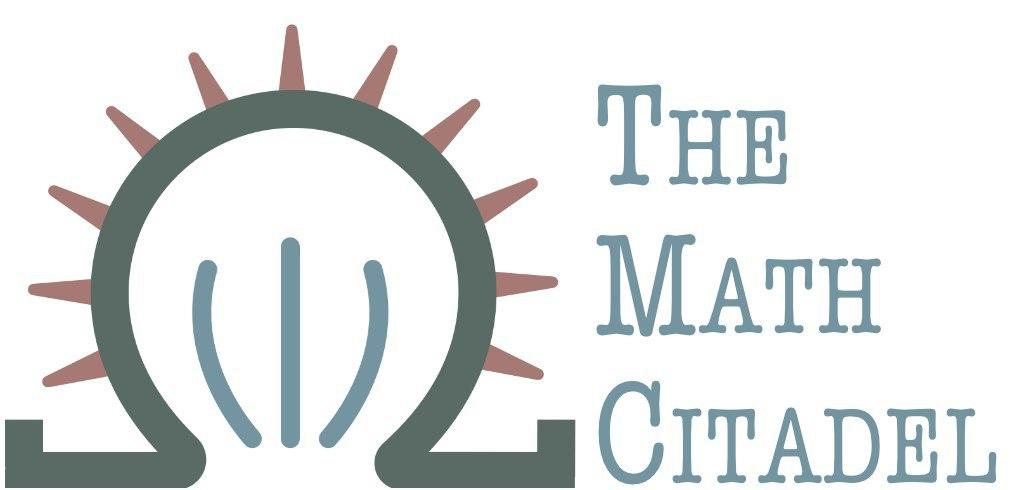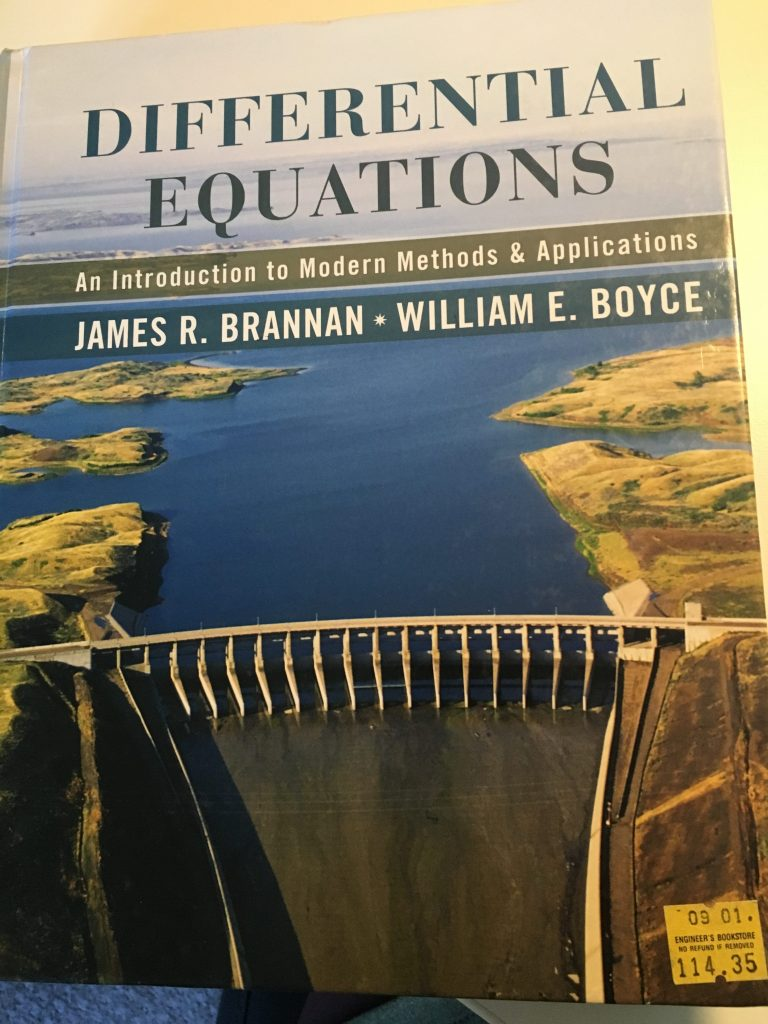## Book Review: Differential Equations: An Introduction to Modern Methods and Applications

### Author: J. Brannan and W. Boyce

#### Reviewed by: R. Traylor## Ranking

All ranking is done on a scale of 1-5, with 5 as the best.
Attribute Rank
Difficulty 3
Proof Quality NA
Self-Study 3
Good for Teaching? 5
Quality of Exercises 5

## Topics Covered

• First order differential equations
• separable equations
• integrating factors
• numerical methods
• systems of two first order equations
• systems of linear algebraic equations
• homogenous linear systems with constant coefficients
• complex/repeated eigenvalues
• second order linear equations
• characteristic equations
• applications in physics
• variation of parameters
• Laplace transform
• convolutions and applications
• systems of first order linear equations
• fundamental matrices
• matrix exponentials
• non homogenous systems
• nonlinear differential equations and stability
• predator-prey equations
• Lorenz equations